# Python Program to Insert an Element in a List

This article is created to cover some programs in Python that insert an element (or more) in a list at the end or at any particular index (or position). Both elements and lists must be entered by the user at runtime. Here is a list of programs:

• Insert an element at the end of a list.
• Insert multiple elements at the end of a list.
• Insert an element at a specific (particular) position in a list.
• Insert multiple elements at some specified indexes in a list.

## Insert an element at the end of a list

This program inserts an element entered by the user at the end of a list. The question is: write a Python program to insert an element in a list. Here is its answer:

```print("Enter 10 Elements of List: ")
nums = []
for i in range(10):
nums.insert(i, input())
print("Enter an Element to Insert at End: ")
elem = input()
nums.append(elem)
print("\nThe New List is: ")
print(nums)```

Here is its sample run:Now supply the input, say 1, 2, 3, 4, 5, 6, 7, 8, 9, 10 as ten numbers, then 11 as a number to insert in the list: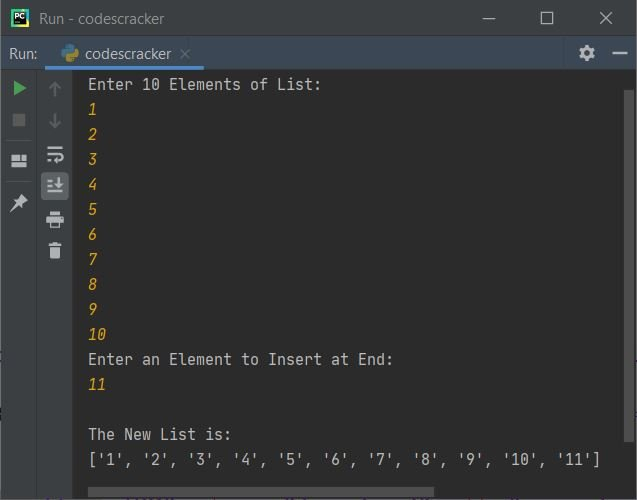In the above program, the following statement:

`nums.insert(i, input())`

states that the entered value gets initialized at ith index of nums. For example, if the user enters 10 and the current value of i is 2, then 10 gets initialized to nums. Since initially the list nums is empty, you can also replace the above statement with statement given below:

`nums.append(input())`

Note: The append() method appends an element (provided as its argument) at the end of a list.

Note: An empty list can also be defined using nums = list().

#### Modified Version of the Previous Program

This program allows the user to define the size of the list. The end used in this program is to skip inserting an automatic newline. str() converts any type of value to a string.

```print("Enter the Size: ", end="")
try:
numsSize = int(input())
print("Enter " +str(numsSize)+ " Elements: ", end="")
nums = []
for i in range(numsSize):
nums.append(input())
print("\nEnter an Element to Insert at End: ")
elem = input()
nums.append(elem)
numsSize = numsSize+1
print("\nThe New List is: ")
for i in range(numsSize):
print(end=nums[i] + " ")
print()
except ValueError:
print("\nInvalid Input!")```

Here is its sample run with user input: 5 as size, 1, 2, 3, 4, 5 as five elements, and 2 as an element to insert at the end of the given list: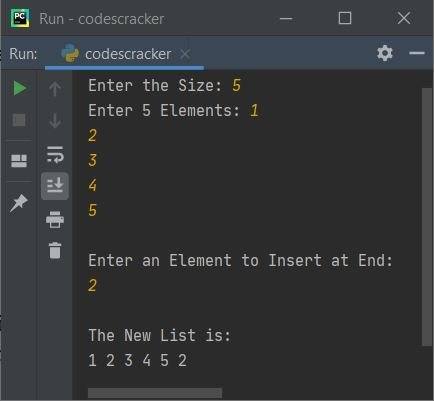Here is another sample run with user input: 11 as size, c, o, d, e, s, c, r, a, c, k, e as eleven elements, and then r as an element to insert at the end of the list: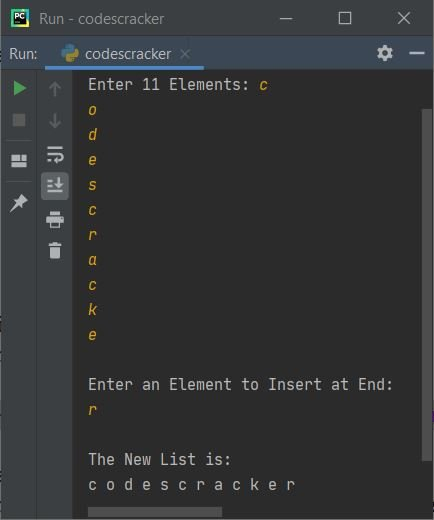From the above program, the following statement:

`print(end=nums[i] + " ")`

can also be written as:

`print(nums[i], end=" ")`

Note: The try-except is used to handle invalid inputs. The first statement of try, numsSize = int(input()), gets executed because it wants to receive an integer input from the user. But if the user enters a non-integer value, then it raises a ValueError; therefore, program flow goes to except ValueError's body and prints a message, whatever the programmer specified.

## Insert Multiple Elements at the End of a List

This program allows the user to insert multiple (more than one) elements at the end of a list during one execution of the program. Let's have a look at the program first:

```print("Enter Size: ", end="")
try:
tot = int(input())
print("Enter " +str(tot)+ " Elements: ", end="")
arr = list()
for i in range(tot):
arr.append(input())
print("\nThe List is:")
for i in range(len(arr)):
print(arr[i], end=" ")
print("\n\nHow many element to insert ? ", end="")
try:
toti = int(input())
print("Enter " +str(toti)+ " Elements: ", end="")
for i in range(toti):
arr.append(input())
print("\nThe New List is:")
for i in range(len(arr)):
print(arr[i], end=" ")
print()
except ValueError:
print("\nInvalid Input!")
except ValueError:
print("\nInvalid Input!")```

Here is its sample run with user inputs: 3 as list size, 1, 2, and 3 as three elements, then 4 as the size of elements to insert, and then 5, 6, 7, and 8 as four elements to insert: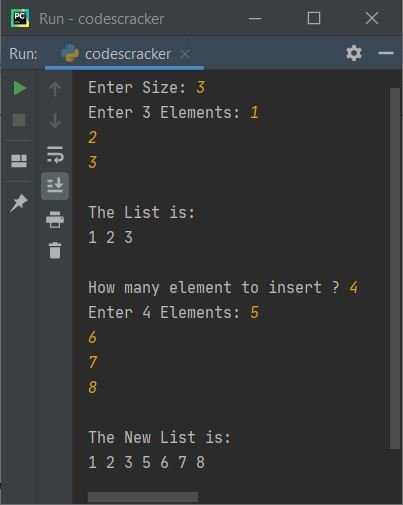The dry run of the above program with the same user input as provided in the sample run given above goes like this:

• When user enters the size say 3, it gets stored in tot variable. So tot=3
• Now using for loop, the following statement:
`arr.append(input())`
gets executed 3 times. So three values gets received using input(), one by one and initialized to arr. For example, if user enters 1, 2, 3 as three elements, then arr = ['1', '2', '3']
• Anything received using input() method, treated as a string type value by default
• Now using another for loop, the elements of list named arr gets displayed
• Then using input(), another value gets received from user as total number of elements to insert
• For example if user enters 4 as input, then 4 gets initialized to toti. So toti=4
• And using another for loop, the statement:
`arr.append(input())`
gets executed four times
• In this way, another four elements gets appended to the list named arr. Because, we're using append() method here, therefore element gets added at the end of list
• In this way, multiple elements gets inserted in the list

## Insert an element at a specific index (position)

As you can see from the very first program of this article, the insert() method is used to insert an element at a particular index number specified as its first argument. Therefore, using the insert() method, this program inserts an element at a specified index by the user at run-time:

```nums = []
print(end="Enter the Size: ")
numsSize = int(input())
print(end="Enter " +str(numsSize)+ " Elements: ")
for i in range(numsSize):
nums.append(input())
print("\nEnter an Element to Insert: ")
elem = input()
print(end="At what index ? ")
pos = int(input())
nums.insert(pos, elem)
numsSize = numsSize+1
print("\nThe New List is: ")
for i in range(numsSize):
print(end=nums[i] + " ")
print()```

Here is its sample run with user input: 5 as the size of the list, 1, 3, 4, 5, 6 as the number of elements, 2 as the element to insert, and 1 as the index of the element to store in the list: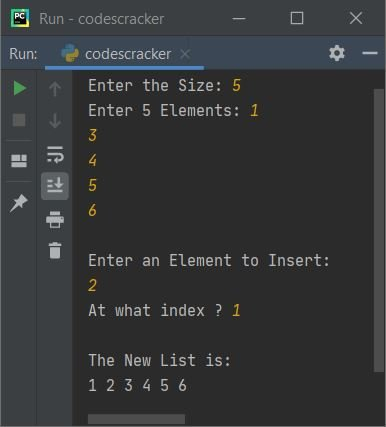Note: To follow the position's value, use the nums.insert((pos+1), elem) statement to insert an element at the given position. Because indexing starts with 0, generally people refer to the 0th index element as the first position's element.

## Insert multiple elements at given indexes in the list

This is the last program in this article, which is a complete version of inserting multiple elements at given indexes in a list at one execution. This program also handles all types of invalid inputs using try-except:

```print("Enter Size: ", end="")
try:
tot = int(input())
print("Enter " +str(tot)+ " Elements: ", end="")
arr = list()
for i in range(tot):
arr.append(input())

print("\nThe List is:")
for i in range(len(arr)):
print(arr[i], end=" ")

print("\n\nHow many element to insert ? ", end="")
try:
toti = int(input())
arrdele = list()
arrdeli = list()

for i in range(toti):
print("Enter Element and its Index Number: ", end="")
arrdele.append(input())
try:
k = int(input())
if k > (len(arr) + len(arrdele)):
print("\nInvalid Input!")
exit()
arrdeli.append(k)
except ValueError:
print("\nInvalid Input!")
exit()

for i in range(toti):
arr.insert(arrdeli[i], arrdele[i])
print("\nThe New List is:")
for i in range(len(arr)):
print(arr[i], end=" ")
print()

except ValueError:
print("\nInvalid Input!")
except ValueError:
print("\nInvalid Input!")```

Here is its sample run with user inputs: 3 as size, 1, 2, 3 as its three elements, and 4 as the number of elements to insert in the entered list right now. Then 11 is the first element, and 0 is its index number. Again, 22 as the second element and 2 as its index number, then 33 as the third element and 4 as its index number, and finally 44 as the element and 6 as its index number: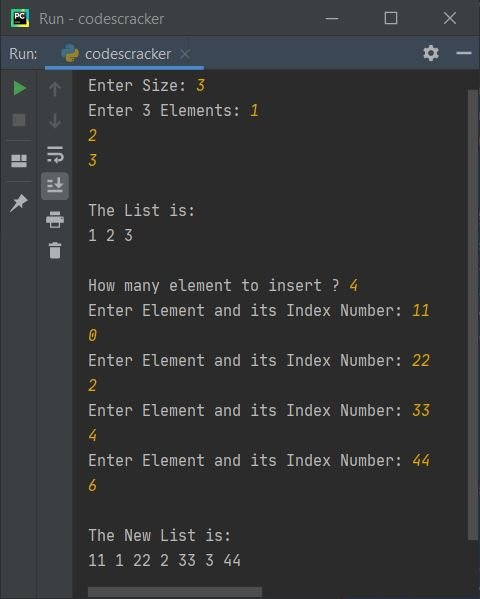#### A Brief Description of the Above Program

The program is created in a way that:

• I've received elements of given size to the list named arr like done previously in above programs
• Then later on, I've used two new lists namely arrdele and arrdeli
• In first list, I've stored all elements that has to be delete. Whereas in second list, I've stored index numbers corresponding to each elements stored in first list
• Then using insert(), I've provided these two lists with its indexes to give index number and element to insert in the list arr
• That is, the statement, arr.insert(arrdeli[i], arrdele[i]), states that the element at ith index of list named arrdeli treated as current index number of arr and element at same index of list named arrdele treated as value to insert in same list. Because I've stored index numbers in list arrdeli, whereas stored elements in list arrdele

The dry run with exactly the same user input as provided in the previous sample run goes like this: This is a value evaluation dry run. The output statement does not involve this dry run:

• tot = 3
• arr = ['1', '2', '3']
• toti = 4
• arrdele = 11
• arrdeli = 0
• arrdele = 22
• arrdeli = 2
• arrdele = 33
• arrdeli = 4
• arrdele = 44
• arrdeli = 6
• Finally, arrdele = [11, 22, 33, 44] and arrdeli = [0, 2, 4, 6]
• Now the main code of insertion, that is:
```for i in range(toti): arr.insert(arrdeli[i], arrdele[i])```
begins its execution
• So at first execution, i=0
arr.insert(arrdeli, arrdele) or arr.insert(0, 11) or arr = '11'. Here 0 and 11 are elements of 0th index of both lists, that is arrdeli and arrdele
• So now arr = ['11', '1', '2', '3']
• At second execution, i=1
arr.insert(arrdeli, arrdele) or arr.insert(2, 22) or arr = '22'
• So now arr = ['11', '1', '22', '2', '3']
• At third execution, i=2
arr.insert(arrdeli, arrdele) or arr.insert(4, 33) or arr = '33'
• So now arr = ['11', '1', '22', '2', '33', '3']
• Similar thing happened with fourth execution
• In this way, multiple elements gets inserted in a list at given indexes.

Python Online Test

« Previous Program Next Program »